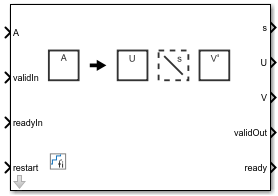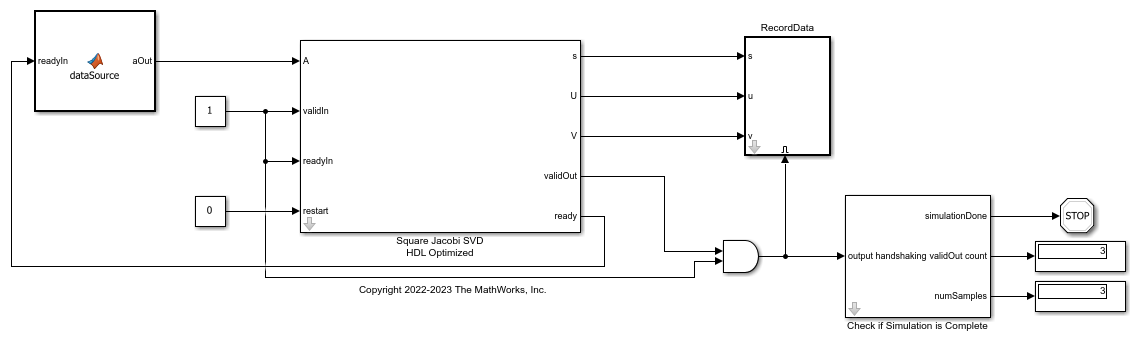# Square Jacobi SVD HDL Optimized

Fixed-point singular value decomposition

Since R2023a

•Libraries:
Fixed-Point Designer HDL Support / Matrices and Linear Algebra / Matrix Factorizations

## Description

Use the Square Jacobi SVD HDL Optimized block to perform singular value decomposition (SVD) on square matrices using the two-sided Jacobi algorithm. Given a square matrix A, the Square Jacobi SVD HDL Optimized block uses the two-sided Jacobi method to produce a vector s of nonnegative elements and unitary matrices U and V such that ```A = U*diag(s)*V'```.

Note

For non-square matrices, use the Non-Square Jacobi SVD HDL Optimized block.

## Examples

expand all

This example shows how to use the Square Jacobi SVD HDL Optimized block to compute the singular value decomposition (SVD) of square matrices.

Two-Sided Jacobi SVD

The Square Jacobi HDL Optimized block uses the two-sided Jacobi algorithm to perform singular value decomposition. Given an input square matrix `A`, the block first computes the two-by-two SVD for off-diagonal elements, then applies the rotation to the `A`, `U`, and `V` matrices. Because the Jacobi algorithm can perform such computations in parallel, it is suitable for FPGA and ASIC applications. For more information, see Square Jacobi SVD HDL Optimized.

Define Simulation Parameters

Specify the dimension of the sample matrices, the number of input sample matrices, and the number of iterations of the Jacobi algorithm.

```n = 8; numSamples = 3; nIterations = 10; ```

Generate Input `A` Matrices

Use the specified simulation parameters to generate the input matrix `A`.

```rng('default'); A = randn(n,n,numSamples); ```

The Square Jacobi SVD HDL Optimized block supports both real and complex inputs. Set the complexity of the input in the block mask accordingly.

```% A = complex(randn(n,n,numSamples),randn(n,n,numSamples)); ```

Select Fixed-Point Data Types

Define the desired word length.

```wordLength = 32; ```

Use the upper bound on the singular values to define fixed-point types that will never overflow. First, use the `fixed.singularValueUpperBound` function to determine the upper bound on the singular values.

```svdUpperBound = fixed.singularValueUpperBound(n,n,max(abs(A(:)))); ```

Define the integer length based on the value of the upper bound, with one additional bit for the sign, another additional bit for intermediate CORDIC growth, and one more bit for intermediate growth to compute the Jacobi rotations.

```additionalBitGrowth = 3; integerLength = ceil(log2(svdUpperBound)) + additionalBitGrowth; ```

Compute the fraction length based on the integer length and the desired word length.

```fractionLength = wordLength - integerLength; ```

Define the signed fixed-point data type to be `'Fixed'` or `'ScaledDouble'`. You can also define the type to be `'double'` or `'single'`.

```dataType = 'Fixed'; T.A = fi([],1,wordLength,fractionLength,'DataType',dataType); disp(T.A) ```
```[] DataTypeMode: Fixed-point: binary point scaling Signedness: Signed WordLength: 32 FractionLength: 24 ```

Cast the matrix `A` to the signed fixed-point type.

```A = cast(A,'like',T.A); ```

Configure Model Workspace and Run Simulation

```model = 'SquareJacobiSVDModel'; open_system(model); fixed.example.setModelWorkspace(model,'A',A,'n',n,... 'nIterations',nIterations,'numSamples',numSamples); out = sim(model); ```Verify Output Solutions

Verify the output solutions. In these steps, "identical" means within roundoff error.

1. Verify that `U*diag(s)*V'` is identical to `A`. `relativeErrorUSV` represents the relative error between `U*diag(s)*V'` and `A`.

2. Verify that the singular values `s` are identical to the floating-point SVD solution. `relativeErrorS` represents the relative error between `s` and the singular values calculated by the MATLAB® `svd` function.

3. Verify that `U` and `V` are unitary matrices. `relativeErrorUU` represents the relative error between `U'*U` and the identity matrix. `relativeErrorVV` represents the relative error between `V'*V` and the identity matrix.

```for i = 1:numSamples disp(['Sample #',num2str(i),':']); a = A(:,:,i); U = out.U(:,:,i); V = out.V(:,:,i); s = out.s(:,:,i); % Verify U*diag(s)*V' if norm(double(a)) > 1 relativeErrorUSV = norm(double(U*diag(s)*V')-double(a))/norm(double(a)); else relativeErrorUSV = norm(double(U*diag(s)*V')-double(a)); end relativeErrorUSV %#ok % Verify s s_expected = svd(double(a)); normS = norm(s_expected); relativeErrorS = norm(double(s) - s_expected); if normS > 1 relativeErrorS = relativeErrorS/normS; end relativeErrorS %#ok % Verify U'*U U = double(U); UU = U'*U; relativeErrorUU = norm(UU - eye(size(UU))) %#ok % Verify V'*V V = double(V); VV = V'*V; relativeErrorVV = norm(VV - eye(size(VV))) %#ok disp('---------------'); end ```
```Sample #1: relativeErrorUSV = 4.5531e-06 relativeErrorS = 2.2997e-06 relativeErrorUU = 5.9074e-07 relativeErrorVV = 6.5182e-07 --------------- Sample #2: relativeErrorUSV = 5.5444e-06 relativeErrorS = 2.2847e-06 relativeErrorUU = 5.7446e-07 relativeErrorVV = 5.2001e-07 --------------- Sample #3: relativeErrorUSV = 5.5542e-06 relativeErrorS = 2.9632e-06 relativeErrorUU = 5.3812e-07 relativeErrorVV = 5.6920e-07 --------------- ```

## Ports

### Input

expand all

Square `n`-by-`n` matrix A, specified as a real or complex square matrix.

If the matrix A has a fixed-point data type, the matrix must use signed binary-point scaling. Slope and bias scaling and unsigned fixed-point types are not supported.

Data Types: `single` | `double` | `fixed point`

Whether input is valid, specified as a Boolean scalar. This control signal indicates when the data from the `A` input port is valid. When this value is `1` (`true`) and the value at `ready` is `1` (`true`), the block captures the values at the `A` input port. When this value is `0` (`false`), the block ignores the input samples.

After sending a `true` `validIn` signal, a delay may occur before the `ready` signal is `false`. To ensure all data is processed, you must wait until the `ready` signal is `false` before sending another `true` `validIn` signal.

Data Types: `Boolean`

Whether downstream block is ready, specified as a `Boolean` scalar. This control signal monitors the `ready` port of the downstream block. When the `readyIn` value is `1` (`true`), and the value at `validOut` is `1` (`true`), the block outputs data to the downstream block. When the `readyIn` value is `0` (`false`), the downstream block is not ready to accept data. The Square Jacobi SVD HDL Optimized block pauses on the output stage and the `ready` signal remains `0` (`false`) until the `readyIn` signal is high.

Data Types: `Boolean`

Whether to clear internal states, specified as a Boolean scalar. When this value is 1 (`true`), the block stops the current calculation and clears all internal states. When this value is 0 (`false`) and the `validIn` value is 1 (`true`), the block begins a new subframe.

Data Types: `Boolean`

### Output

expand all

Singular values, returned as a column vector of length `n`. The Singular Values are nonnegative and returned in descending order such that `s(1) >= s(2) >= ... >= s(n)`. Singular values are returned with the same data type as the input matrix `A`.

Data Types: `single` | `double` | `fixed point`

Left singular vectors, returned as a unitary `n`-by-`n` matrix.

For fixed-point and scaled-double inputs, `U` is returned as a signed fixed-point or scaled-double `fi` with the same word length as `A` and fraction length equal to two less than the word length. One of these integer bits is used for the sign. The other integer bit allows `+1` to be represented exactly.

For floating-point input, `U` has the same data type as `A`.

Data Types: `single` | `double` | `fixed point`

Right singular vectors, returned as a unitary `n`-by-`n` matrix.

For fixed-point and scaled-double inputs, `V` is returned as a signed fixed-point or scaled-double `fi` with the same word length as `A` and fraction length equal to two less than the word length. One of these integer bits is used for the sign. The other integer bit allows `+1` to be represented exactly.

For floating-point input, `V` has the same data type as `A`.

Data Types: `single` | `double` | `fixed point`

Whether the output data is valid, returned as a Boolean scalar. This control signal indicates when the data at the output ports `U`, `S`, and `V` are valid. When this value is `1` (`true`), the output data is valid. When this value is `0` (`false`), the output data is not valid. Transfer of data to the downstream block occurs only when both the `validOut` and `readyIn` signals are high.

Data Types: `Boolean`

Whether the block is ready, returned as a Boolean scalar. This control signal indicates when the block is ready for new input data. When this value is `1` (`true`) and the `validIn` value is `1` (`true`), the block accepts input data in the next time step. When this value is `0` (`false`), the block ignores input data in the next time step.

After sending a `true` `validIn` signal, there may be some delay before the `ready` signal is `false`. To ensure all data is processed, you must wait until the `ready` signal is `false` before sending another `true` `validIn` signal.

Data Types: `Boolean`

## Parameters

expand all

Dimension of square matrix A, specified as a positive integer-valued scalar.

#### Programmatic Use

 Block Parameter: `n` Type: `string` Values: positive integer-valued scalar Default: `n`

Number of iterations of the Jacobi algorithm, specified as a positive integer. Most sources indicate that 10 iterations is sufficient for the Jacobi algorithm to converge .

#### Programmatic Use

 Block Parameter: `nIterations` Type: `string` Values: positive integer-valued scalar Default: `10`

Block outputs, specified as `UsV`, `Us`, `sV`, or `s`.

#### Programmatic Use

 Block Parameter: `outputOption` Type: `string` Values: `UsV` | `Us` | `sV` | `s` Default: `UsV`

Complexity of the input matrix A, specified as `real`, or `complex`.

#### Programmatic Use

 Block Parameter: `complexity` Type: `string` Values: `real` | `complex` Default: `real`

## Tips

• The Square Jacobi SVD HDL Optimized block computes the singular value decomposition in place. Set the fixed-point data type of the input `n`-by-`n` matrix `A` with enough precision and enough headroom to avoid overflow.

First, use the `fixed.singularValueUpperBound` function to determine the upper bound on the singular values. Then define the integer length based on the value of the upper bound, with one additional bit for the sign, another additional bit for intermediate CORDIC growth, and one more bit for intermediate growth to compute the Jacobi rotations. Compute the fraction length based on the integer length and the desired word length.

```svdUpperBound = fixed.singularValueUpperBound(n,n,max(abs(A(:)))) additionalBitGrowth = 3; integerLength = ceil(log2(svdUpperBound)) + additionalBitGrowth wordLength = 16 fractionLength = wordLength - integerLength```
• The behavior of the Square Jacobi SVD HDL Optimized block is equivalent to `[U,s,V] = fixed.jacobiSVD(A)`. The `fixed.JacobiSVD` function uses the same algorithm as the Square Jacobi SVD HDL Optimized, with the same output data types. However, there may be small numerical differences in the least-significant bit between the function and the block.

• The behavior of the Square Jacobi SVD HDL Optimized block is equivalent to `[U,s,V] = fixed.svd(A,'econ','vector')` when `A` is a square matrix.

## Algorithms

expand all

 Jacobi, Carl G. J., "Über ein leichtes Verfahren die in der Theorie der Säcularstörungen vorkommenden Gleichungen numerisch aufzulösen." Journal fur die reine und angewandte Mathematik 30 (1846): 51–94.

 Forsythe, George E. and Peter Henrici. "The Cyclic Jacobi Method for Computing the Principal Values of a Complex Matrix." Transactions of the American Mathematical Society 94, no. 1 (January 1960): 1-23.

 Shiri, Aidin and Ghader Khosroshahi. "An FPGA Implementation of Singular Value Decomposition", ICEE 2019: 27th Iranian Conference on Electrical Engineering, Yazd, Iran, April 30–May 2, 2019, 416-22, IEEE.

 Golub, Gene H. and Charles F. Van Loan. Matrix Computations, 4th ed. Baltimore, MD: Johns Hopkins University Press, 2013.

 Athi, Mrudula V., Seyed R. Zekavat, and Alan A. Struthers. "Real-Time Signal Processing of Massive Sensor Arrays via a Parallel Fast Converging SVD Algorithm: Latency, Throughput, and Resource Analysis." IEEE Sensors Journal 16, no. 18 (January 2016): 2519-26. https://doi.org/10.1109/JSEN.2016.2517040.

 Brent, Richard P., Franklin T. Luk, and Charles Van Loan. "Computation of the Singular Value Decomposition Using Mesh-Connected Processors." Journal of VLSI and Computer Systems 1, 3 (1985): 242–70.

 Hemkumar, Nariankadu D. A Systolic VLSI Architecture for Complex SVD. Master’s thesis, Rice University, 1991.

 Duryea, R. A. Finite Precision Arithmetic in Singular Value Decomposition Architectures. Ph.D. thesis, Cornell University, 1987.

 Cavallaro, Joseph R. and Franklin T. Luk. 1987. "CORDIC Arithmetic for an SVD Processor." 1987 IEEE 8th Symposium on Computer Arithmetic (ARITH), Como, Italy, May 18-21, 1987, 113-20. IEEE. https://doi.org/10.1109/ARITH.1987.6158686.